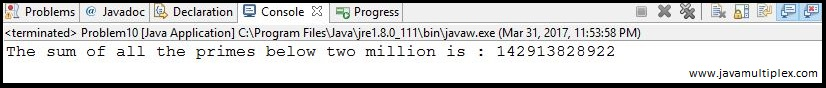## Problem Description :

The sum of the primes below
10 is 2 + 3 + 5 + 7 = 17. Find the sum of all the primes below two million.

## Concept :

For generating prime numbers we are using Sieve of Eratosthenes algorithm. This is very simple and efficient algorithm for generating primes.

## Algorithm :

If you want to generate all prime number below N. Please follow below steps.

• Create boolean array of  length N and assign 'TRUE' to all elements of an array.
• Find the sqrt of N because loop will run from 2 to sqrt(N).
• In loop, check whether element present at current index is 'TRUE'. If yes, then assign 'FALSE' to all elements whose index is multiple of current index.Sieve of Eratosthenes algorithm

Once prime numbers generated, run the loop from 2 to limit(2 million) and check whether element present at current index is prime or not. If element is prime then add that index.

## Output :Output - Project Euler Problem 10 solution in Java

## References :

https://projecteuler.net/problem=10

https://en.wikipedia.org/wiki/Sieve_of_Eratosthenes

http://docs.oracle.com/javase/7/docs/api/java/lang/Math.html

Thank you friends, I hope you have clearly understood the solution of this problem. If you have any doubt, suggestion or query please feel free to comment below. You can also discuss this solution in our forum.

Tags : Project Euler Problem 10 solution in Java, Prime numbers, Summation of primes, Mathematics problems, if else statement, for loop, Eratosthenes Algorithm, Arrays.Project Euler | Problem 10 | Summation of primesReviewed by Rohit Agarwal on 3/31/2017 Rating: 5